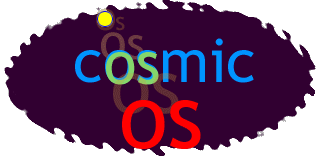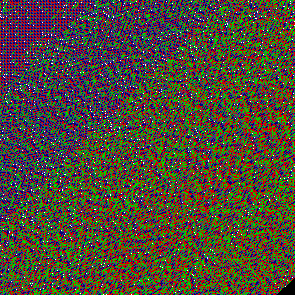# CosmicOS version "cosmic.0.7"

(latest version always available at CosmicOS homepage)

 (version notes at bottom of this page) Estimate of message entropy in final form: 11.4 kB. There are bugs and rough edges in the message. Please be forgiving. It'll all get fixed in an instant of galactic time...

 Message index
(complete message)

 0 introduce numbers (in unary notation) (MATH) 1 introduce equality for unary numbers (MATH) 2 now introduce other relational operators (MATH) 3 introduce tail notation (SYNTAX) 4 introduce the NOT logical operator (MATH) 5 introduce the AND logical operator (MATH) 6 introduce the OR logical operator (MATH) 7 use equality for truth values (MATH) 8 introduce addition (MATH) 9 introduce subtraction (MATH) 10 introduce multiplication (MATH) 11 introduce a simple form of binary notation (MATH) 12 demonstrate idea of leaving gaps in an expression (MATH) 13 show some simple function calls (MATH) 14 show mechanisms for branching (MATH) 15 some pure lambda calculus definitions - optional (MATH) 16 show an example of recursive evaluation (MATH) 17 introduce universal quantifier (MATH) 18 introduce existential quantifier (MATH) 19 introduce logical implication (MATH) 20 illustrate lists and some list operators (MATH) 21 describe changes to the implicit interpreter to allow new special forms (HACK) 22 introduce sugar for let (MATH) 23 build up functions of several variables (MATH) 24 show map function for applying a function across the elements of a list (MATH) 25 introduce mutable objects, and side-effects (MATH) 26 show how to execute a sequence of instructions (MATH) 27 introduce environment / hashmap structure (MATH) 28 introduce simple mutable structures (OBJECT) 29 introduce method handler wrappers (OBJECT) 30 introduce turing machine model (TURING) 31 introduce sets and set membership (MATH) 32 introduce graph structures (MATH) 33 introduce simple form of typing, for ease of documentation. (OBJECT) 34 an example object -- a 2D point (OBJECT) 35 an example object -- a container (OBJECT) 36 expressing inheritance (OBJECT) 37 adding a special form for classes (OBJECT) 38 wrapper class for cells (OBJECT) 39 playing around with doors and rooms (MUD) 40 some preparatory work for integrating with Java code (JAVA) 41 class translation 'COS_JavaTest' (JAVA) 42 check that automatic conversion is workable (JAVA)

 Message visualization

 message visualized as imageThe image reads diagonally, starting at the top left. It is handy for spotting phase changes in the message (e.g. the transition from using unary to binary, or - more importantly - bugs). Breaks between statements are white dots, zeros are greenish, ones are reddish, parentheses are different shades of blue.

 Version notes

The advantage of using a programming-code-like language is that the reader can play with hypothethicals at any time, and experiment to evaluate alternative statements that are not in the message.

Current status: Playing with integrating the Java virtual machine. Looks doable, and Java bytecode can be generated now from many languages (including Scheme). Also trying to make the core functional notation more readable. It is in transition, and some things are broken.

Functions currently introduced through examples, rather than completely defined in terms of other functions:

The generated message currently consists of a sequence of 6 symbols.

```  number   symbol   meaning
0         .     binary digit zero
1         :     binary digit one
2         (     marks beginning of an expression
3         )     marks end of an expression
4         /     opens an implicit paren, which will close at next paren
5         ;     marks end of sentence
```

There are constraints in the possible transitions between these symbols that would allow a simple and shorter encoding if desired.

Numbers are encoded as binary digits between parentheses, e.g. (:::.) is 1110 base 2 which is 14 in decimal. A set of numbers between parentheses constitutes an expression. Expressions can be nested. Expressions followed by a semicolon should evaluate to be true, once the rules for evaluation have been introduced.

In the human-readable form of the message, decimal numbers can be used. There are converted to the above form. Identifiers can also be used. Identifiers are mapped onto arbitrarily assigned numbers. In the message, there is nothing to distinguish identifiers from numbers. The actual language is carefully constructed so that this distinction is never necessary.

The first number in an expression is treated as an index into an environment that returns a function. When the lambda notation is introduced, it works by modifying that (nested) environment. Expressions are evaluated from the outermost inwards, from left to right, and the "if" form is introduced as lazy.

This "functional style" of expression is not always particularly easy to follow, even for a human, but it is certainly very expressive. Currently functional definitions are given alongside numerous examples that are in many cases sufficient by themselves to communicate the definition, at least for working purposes. It is probably important to maintain this duality and perhaps extend it with other forms of expression. There are so many models of computation, why not use all of them? Perhaps one will be easier for the reader to follow than the others.

While it is tempting to try to make the message airtight from a formal point of view, defining everything in terms of axioms, this is just one didactic approach - and may be counter-productive or impossible for a a large-scale message that includes AI-complete concepts.

Currently there is a conflict between using definitions of functions that are easy to communicate, and definitions that are efficient (or external). This will require some more thought. For example, it would be nice to introduce "if" in its pure lambda calculus form, but to do so would slow checking down right now. The "if" function is instead built in, and (to add insult to injury) introduced with lazy evaluation. It would be more consistent to keep everything eager to begin with, and then show the evaluator being rewritten to facilitate laziness -- easy using the standard trick of wrapping conditional expressions in single-argument functions.